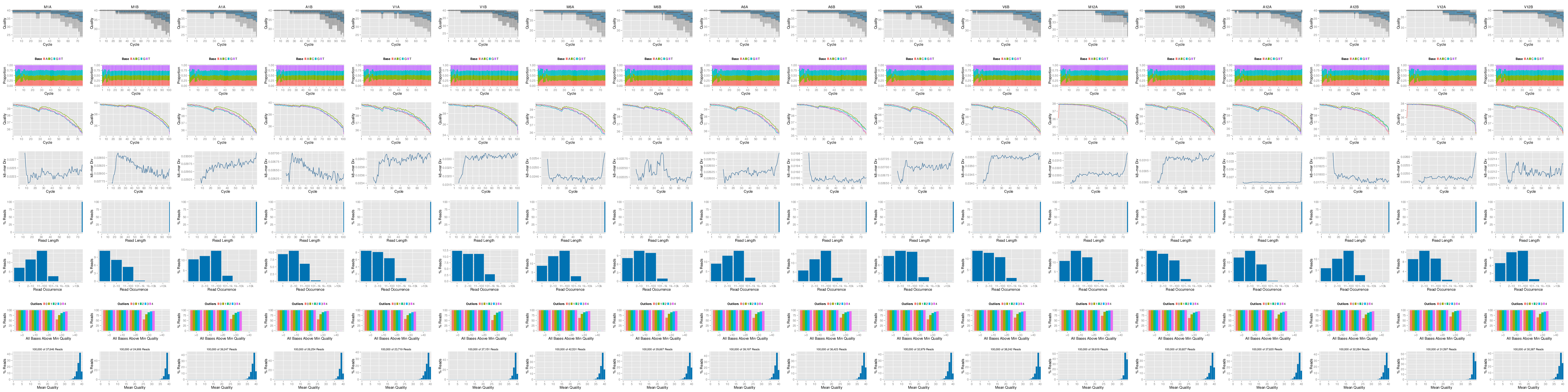**Figure 1:** FASTQ quality report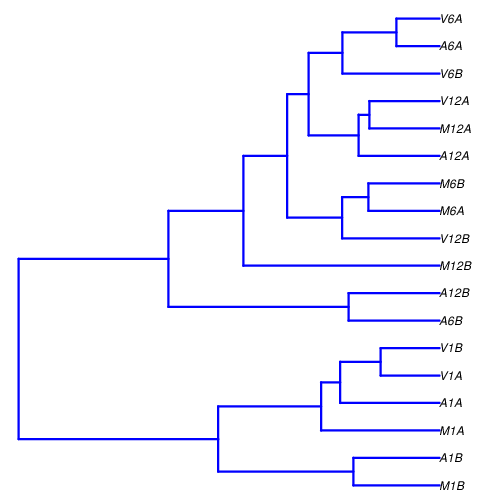**Figure 2:** Correlation dendrogram of samples for _`rlog`_ values.

## DEG analysis with _`edgeR`_ The following _`run_edgeR`_ function is a convenience wrapper for identifying differentially expressed genes (DEGs) in batch mode with _`edgeR`_'s GML method [@Robinson2010-uk] for any number of pairwise sample comparisons specified under the _`cmp`_ argument. Users are strongly encouraged to consult the [_`edgeR`_](\href{http://www.bioconductor.org/packages/devel/bioc/vignettes/edgeR/inst/doc/edgeRUsersGuide.pdf) vignette for more detailed information on this topic and how to properly run _`edgeR`_ on data sets with more complex experimental designs. ```{r edger, message=FALSE, eval=TRUE, spr='r'} appendStep(sal) <- LineWise({ targetspath <- system.file("extdata", "targets.txt", package = "systemPipeR") targets <- read.delim(targetspath, comment = "#") cmp <- readComp(file = targetspath, format = "matrix", delim = "-") countDFeBygpath <- system.file("extdata", "countDFeByg.xls", package = "systemPipeR") countDFeByg <- read.delim(countDFeBygpath, row.names = 1) edgeDF <- run_edgeR(countDF = countDFeByg, targets = targets, cmp = cmp[], independent = FALSE, mdsplot = "") DEG_list <- filterDEGs(degDF = edgeDF, filter = c(Fold = 2, FDR = 10)) }, step_name = "edger", dependency = "read_counting" ) ``` Filter and plot DEG results for up and down-regulated genes. Because of the small size of the toy data set used by this vignette, the _FDR_ value has been set to a relatively high threshold (here 10%). More commonly used _FDR_ cutoffs are 1% or 5%. The definition of '_up_' and '_down_' is given in the corresponding help file. To open it, type _`?filterDEGs`_ in the R console.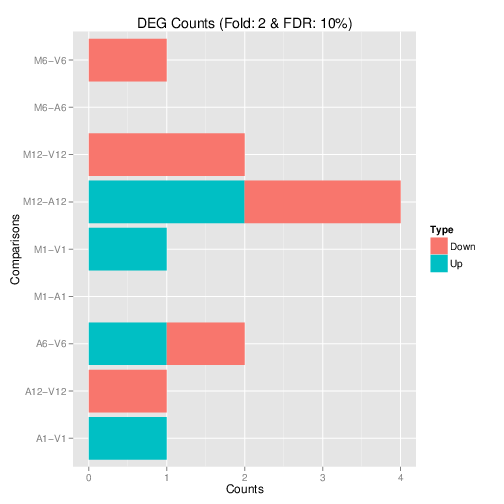**Figure 3:** Up and down regulated DEGs identified by _`edgeR`_.

## DEG analysis with _`DESeq2`_ The following _`run_DESeq2`_ function is a convenience wrapper for identifying DEGs in batch mode with _`DESeq2`_ [@Love2014-sh] for any number of pairwise sample comparisons specified under the _`cmp`_ argument. Users are strongly encouraged to consult the [_`DESeq2`_](http://www.bioconductor.org/packages/devel/bioc/vignettes/DESeq2/inst/doc/DESeq2.pdf) vignette for more detailed information on this topic and how to properly run _`DESeq2`_ on data sets with more complex experimental designs. ```{r deseq2, message=FALSE, eval=TRUE, spr='r'} appendStep(sal) <- LineWise({ degseqDF <- run_DESeq2(countDF=countDFeByg, targets=targets, cmp=cmp[], independent=FALSE) DEG_list2 <- filterDEGs(degDF=degseqDF, filter=c(Fold=2, FDR=10)) }, step_name = "deseq2", dependency = "read_counting" ) ``` ## Venn Diagrams The function _`overLapper`_ can compute Venn intersects for large numbers of sample sets (up to 20 or more) and _`vennPlot`_ can plot 2-5 way Venn diagrams. A useful feature is the possibility to combine the counts from several Venn comparisons with the same number of sample sets in a single Venn diagram (here for 4 up and down DEG sets). ```{r vennplot, message=FALSE, eval=TRUE, spr='r'} appendStep(sal) <- LineWise({ vennsetup <- overLapper(DEG_list\$Up[6:9], type="vennsets") vennsetdown <- overLapper(DEG_list\$Down[6:9], type="vennsets") vennPlot(list(vennsetup, vennsetdown), mymain="", mysub="", colmode=2, ccol=c("blue", "red")) }, step_name = "vennplot", dependency = "edger" ) ```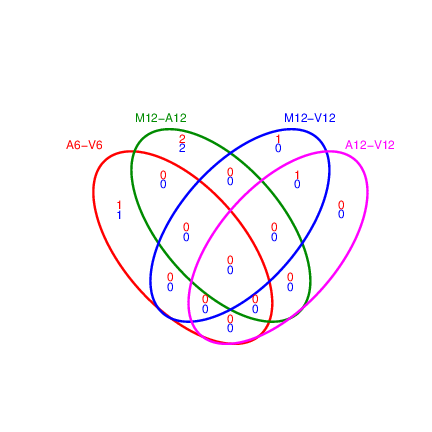**Figure 4:** Venn Diagram for 4 Up and Down DEG Sets.

## GO term enrichment analysis of DEGs ### Obtain gene-to-GO mappings The following shows how to obtain gene-to-GO mappings from _`biomaRt`_ (here for _A. thaliana_) and how to organize them for the downstream GO term enrichment analysis. Alternatively, the gene-to-GO mappings can be obtained for many organisms from Bioconductor's _`*.db`_ genome annotation packages or GO annotation files provided by various genome databases. For each annotation, this relatively slow preprocessing step needs to be performed only once. Subsequently, the preprocessed data can be loaded with the _`load`_ function as shown in the next subsection. ```{r get_go_biomart, message=FALSE, eval=TRUE, spr='r'} appendStep(sal) <- LineWise({ library("biomaRt") listMarts() # To choose BioMart database listMarts(host="plants.ensembl.org") m <- useMart("plants_mart", host="https://plants.ensembl.org") listDatasets(m) m <- useMart("plants_mart", dataset="athaliana_eg_gene", host="https://plants.ensembl.org") listAttributes(m) # Choose data types you want to download go <- getBM(attributes=c("go_id", "tair_locus", "namespace_1003"), mart=m) go <- go[go[,3]!="",]; go[,3] <- as.character(go[,3]) go[go[,3]=="molecular_function", 3] <- "F" go[go[,3]=="biological_process", 3] <- "P" go[go[,3]=="cellular_component", 3] <- "C" go[1:4,] dir.create("./data/GO") write.table(go, "data/GO/GOannotationsBiomart_mod.txt", quote=FALSE, row.names=FALSE, col.names=FALSE, sep="\t") catdb <- makeCATdb(myfile="data/GO/GOannotationsBiomart_mod.txt", lib=NULL, org="", colno=c(1,2,3), idconv=NULL) save(catdb, file="data/GO/catdb.RData") }, step_name = "get_go_biomart", dependency = "edger" ) ``` ### Batch GO term enrichment analysis Apply the enrichment analysis to the DEG sets obtained in the above differential expression analysis. Note, in the following example the _FDR_ filter is set here to an unreasonably high value, simply because of the small size of the toy data set used in this vignette. Batch enrichment analysis of many gene sets is performed with the _`GOCluster_Report`_ function. When _`method="all"`_, it returns all GO terms passing the p-value cutoff specified under the _`cutoff`_ arguments. When _`method="slim"`_, it returns only the GO terms specified under the _`myslimv`_ argument. The given example shows how one can obtain such a GO slim vector from BioMart for a specific organism. ```{r go_enrichment, message=FALSE, eval=TRUE, spr='r'} appendStep(sal) <- LineWise({ load("data/GO/catdb.RData") DEG_list <- filterDEGs(degDF=edgeDF, filter=c(Fold=2, FDR=50), plot=FALSE) up_down <- DEG_list\$UporDown; names(up_down) <- paste(names(up_down), "_up_down", sep="") up <- DEG_list\$Up; names(up) <- paste(names(up), "_up", sep="") down <- DEG_list\$Down; names(down) <- paste(names(down), "_down", sep="") DEGlist <- c(up_down, up, down) DEGlist <- DEGlist[sapply(DEGlist, length) > 0] BatchResult <- GOCluster_Report(catdb=catdb, setlist=DEGlist, method="all", id_type="gene", CLSZ=2, cutoff=0.9, gocats=c("MF", "BP", "CC"), recordSpecGO=NULL) library("biomaRt") m <- useMart("plants_mart", dataset="athaliana_eg_gene", host="https://plants.ensembl.org") goslimvec <- as.character(getBM(attributes=c("goslim_goa_accession"), mart=m)[,1]) BatchResultslim <- GOCluster_Report(catdb=catdb, setlist=DEGlist, method="slim", id_type="gene", myslimv=goslimvec, CLSZ=10, cutoff=0.01, gocats=c("MF", "BP", "CC"), recordSpecGO=NULL) gos <- BatchResultslim[grep("M6-V6_up_down", BatchResultslim\$CLID), ] gos <- BatchResultslim pdf("GOslimbarplotMF.pdf", height=8, width=10); goBarplot(gos, gocat="MF"); dev.off() goBarplot(gos, gocat="BP") goBarplot(gos, gocat="CC") }, step_name = "go_enrichment", dependency = "get_go_biomart" ) ``` ### Plot batch GO term results The _`data.frame`_ generated by _`GOCluster_Report`_ can be plotted with the _`goBarplot`_ function. Because of the variable size of the sample sets, it may not always be desirable to show the results from different DEG sets in the same bar plot. Plotting single sample sets is achieved by subsetting the input data frame as shown in the first line of the following example.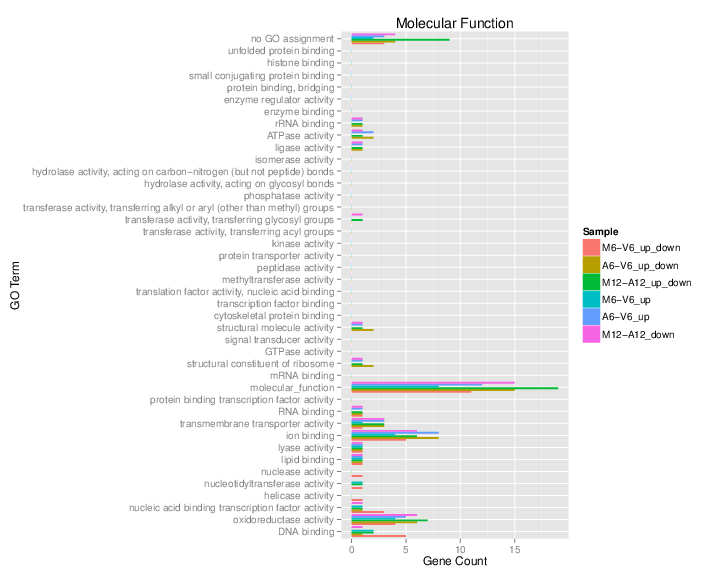**Figure 5:** GO Slim Barplot for MF Ontology.

## Clustering and heat maps The following example performs hierarchical clustering on the _`rlog`_ transformed expression matrix subsetted by the DEGs identified in the above differential expression analysis. It uses a Pearson correlation-based distance measure and complete linkage for cluster join. ```{r hierarchical_clustering, message=FALSE, eval=TRUE, spr='r'} appendStep(sal) <- LineWise({ library(pheatmap) geneids <- unique(as.character(unlist(DEG_list[]))) y <- assay(rlog(dds))[geneids, ] pdf("heatmap1.pdf") pheatmap(y, scale="row", clustering_distance_rows="correlation", clustering_distance_cols="correlation") dev.off() }, step_name = "hierarchical_clustering", dependency = c("sample_tree_rlog", "edger") ) ```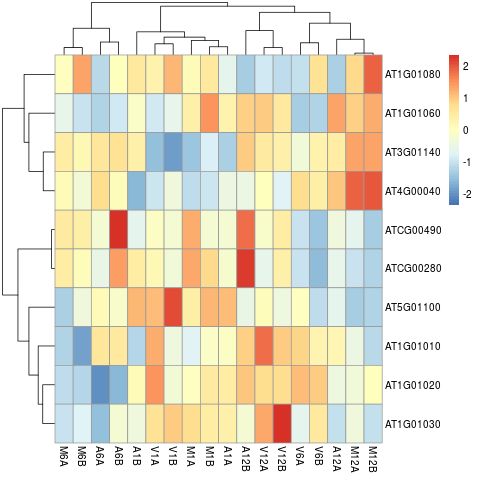**Figure 7:** Heat map with hierarchical clustering dendrograms of DEGs.

## Visualize workflow systemPipeR workflows instances can be visualized with the `plotWF` function. This function will make a plot of selected workflow instance and the following information is displayed on the plot: - Workflow structure (dependency graphs between different steps); - Workflow step status, *e.g.* `Success`, `Error`, `Pending`, `Warnings`; - Sample status and statistics; - Workflow timing: running duration time. If no argument is provided, the basic plot will automatically detect width, height, layout, plot method, branches, etc. ```{r} plotWF(sal, show_legend = TRUE, width = "80%") ``` ## Running the workflow For running the workflow, `runWF` function will execute all the command lines store in the workflow container. ```{r, eval=FALSE} sal <- runWF(sal) ``` #### Interactive job submissions in a single machine To simplify the short read alignment execution for the user, the command-line can be run with the *`runCommandline`* function. The execution will be on a single machine without submitting to a queuing system of a computer cluster. This way, the input FASTQ files will be processed sequentially. By default *`runCommandline`* auto detects SAM file outputs and converts them to sorted and indexed BAM files, using internally the `Rsamtools` package [@Rsamtools]. Besides, *`runCommandline`* allows the user to create a dedicated results folder for each workflow and a sub-folder for each sample defined in the *targets* file. This includes all the output and log files for each step. When these options are used, the output location will be updated by default and can be assigned to the same object. If available, multiple CPU cores can be used for processing each file. The number of CPU cores (here 4) to use for each process is defined in the _`*.yml`_ file. With _`yamlinput(args)['thread']`_ one can return this value from the _`SYSargs2`_ object. #### Parallelization on clusters Alternatively, the computation can be greatly accelerated by processing many files in parallel using several compute nodes of a cluster, where a scheduling/queuing system is used for load balancing. For this the *`clusterRun`* function submits the computing requests to the scheduler using the run specifications defined by *`runCommandline`*. To avoid over-subscription of CPU cores on the compute nodes, the value from _`yamlinput(args)['thread']`_ is passed on to the submission command, here _`ncpus`_ in the _`resources`_ list object. The number of independent parallel cluster processes is defined under the _`Njobs`_ argument. The following example will run 18 processes in parallel using for each 4 CPU cores. If the resources available on a cluster allow running all 18 processes at the same time then the shown sample submission will utilize in total 72 CPU cores. Note, *`clusterRun`* can be used with most queueing systems as it is based on utilities from the _`batchtools`_ package which supports the use of template files (_`*.tmpl`_) for defining the run parameters of different schedulers. To run the following code, one needs to have both a conf file (see _`.batchtools.conf.R`_ samples [here](https://mllg.github.io/batchtools/)) and a template file (see _`*.tmpl`_ samples [here](https://github.com/mllg/batchtools/tree/master/inst/templates)) for the queueing available on a system. The following example uses the sample conf and template files for the Slurm scheduler provided by this package. ## Access the Previous Version Please find [here](/sp/spr/steps_oldversion/) the previous version. ```{r quiting, eval=TRUE, echo=FALSE} knitr::opts_knit\$set(root.dir = '../') unlink("rnaseq", recursive = TRUE) ``` ## References